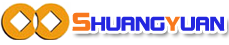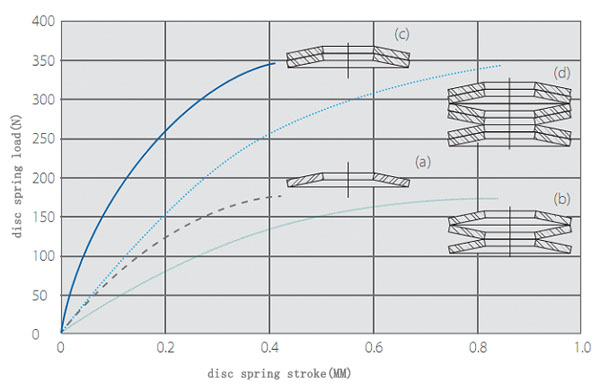# Disc Springs Combination And Curve Relationship

The combination of the disc spring is divided into parallel and series two kinds.
(a) single displacement increase with the load increase
(b) Three piece series the same load, displacement increased by three times
(c) Double parallel the same displacement, the load increases twice times
(d) Three groups of two parallel series load increase twice the displacement of a three-fold increase- - - - (a)single disc spring
── (b)Three piece series,displacement increased by three times ,the load increases twice times
━━ (c)Double parallel ,the load increases twice times
…….. (d)Three groups of two parallel series load increase twice the displacement of a three-fold increase

◆Using combinations of different thickness can make the discs spring characteristic curve into a downward parabola
◆The same size disc spring combination insert different thickness washers or use of the same thickness washer to distinguish between the number of series of different groups can achieve the same effect
◆Free combination, so that application of disc spring wider